# 40% volume

40% volume with 104 uph (units per labor hour) 8 people working. What is the volume?

x =  16640

### Step-by-step explanation:

40/100 • x = 104 • 8 • 8

40x = 665600

x = 16640

Our simple equation calculator calculates it.Did you find an error or inaccuracy? Feel free to write us. Thank you!Tips to related online calculators
Do you have a linear equation or system of equations and looking for its solution? Or do you have a quadratic equation?
Do you want to convert time units like minutes to seconds?

## Related math problems and questions:

• The workThe work was to be done by 150 workers. At the beginning of their work, their number reduced by 40, which increased the time of work by 5 and 1/3 of the schedule. How long did work take?
• Two companies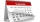The family ordered two companies to modify the garden. The first would be done in 20 days, the second would take 40% less time. How long will they work together if the second company started working when the first worked for 4 days?
• SolutionsHow much 60% solution and how much 35% solution is needed to create 100 l of 40% solution?
• Ditch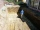The excavator dug 40% of the excavation in 2 hours 20 minutes. How long does it take him to dig the whole ditch?
• WorkshopsThe plant has three workshops. In the first workshop, produce 5 products/hour, in the second 8 products/hour, and in the third 7 products/hour. In the first workshop, they produced 240 products, in the second 400 and the third 350 products. Find the avera
• Two water containers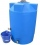In the first container, there are 200 m3 of water and in the second 40 m3. The first container will flow down at a rate of 10 m3 water per hour. At the same time flows to the second rate of 5 m3 per hour. After how many hours there will be three times les
• Alcohol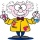6l 48% alcohol, how much 52% alcohol must be added to give 50% alcohol?
• Mukls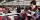The working group, in which 6 workers would ordered job completed within 21 working days. How many workers must be accept yet that the contract will be completed in 14 working days?
• Four numbersThe first number is 50% second, the second number is 40% third, the third number is 20% of the fourth. The sum is 396. What are the numbers?
• Percents from percentHow much is 13% of 20% of 500 greater than 8% of 14% of 200?
• Working aloneTom and Chandri are doing household chores. Chandri can do the work twice as fast as Tom. If they work together, they can finish the work in 5 hours. How long does it take Tom working alone to do the same work?
• Working together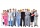Two people will do the work in 12 days. They worked together for 8 days. Then only one worked for 10 days. How many days would each of them do the work if he worked alone?
• Copper sulphate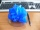How much g of water do we have to add to 240 g of an 84% CuSO4 solution to produce a 60% solution? (Express the mass of crystalline CuSO4 in the original solution and in the resulting solution and compare them. )
• ConcentrationHow many grams of 65% and 50% acid is to be mixed to give 240 g acid with a concentration of 60%?
• Three workshopsThere are 2743 people working in three workshops. In the second workshop works 140 people more than in the first and in third works 4.2 times more than the second one. How many people work in each workshop?
• Beds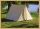At the summer camp, there are 41 chalets. Some rooms are 3-beds, some 4-beds. How many campers from 140 are living in 3-bed?
• Five pupils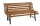Five pupils clean 30 chairs one hour before four pupils. How many chairs clean one pupil in 1 hour?